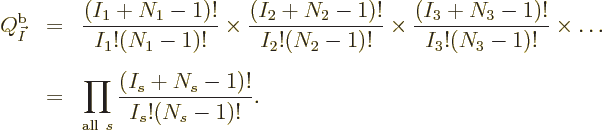### D.56 Num­ber of sys­tem eigen­func­tions

This note de­rives the num­ber of en­ergy eigen­func­tions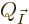for a given set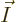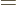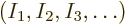of shelf oc­cu­pa­tion num­bers,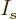be­ing the num­ber of par­ti­cles on shelf num­ber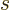. The num­ber of sin­gle-par­ti­cle eigen­func­tions on shelf num­beris in­di­cated by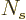.

Con­sider first the case of dis­tin­guish­able par­ti­cles, re­fer­ring to fig­ure 11.1 for an ex­am­ple. The ques­tion is how many dif­fer­ent eigen­func­tions can be cre­ated with the given shelf num­bers. What are the ways to cre­ate dif­fer­ent ones? Well, the first choice that can be made is what are the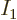par­ti­cles that go on shelf 1. If you pick outpar­ti­cles from the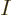to­tal par­ti­cles, you havechoices for par­ti­cle 1, next there are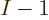choices left for par­ti­cle 2, then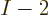for par­ti­cle 3. The to­tal num­ber of pos­si­ble ways of choos­ing thepar­ti­cles is then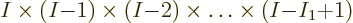How­ever, this over­es­ti­mates the num­ber of vari­a­tions in eigen­func­tions that you can cre­ate by se­lect­ing thepar­ti­cles: the only thing that makes a dif­fer­ence for the eigen­func­tions is what par­ti­cles you pick to go on shelf 1; the or­der in which you chose to pick them out of the to­tal set ofmakes no dif­fer­ence. If you chose a set ofpar­ti­cles in an ar­bi­trary or­der, you get no dif­fer­ence in eigen­func­tion com­pared to the case that you pick out the same par­ti­cles sorted by num­ber. To cor­rect for this, the num­ber of eigen­func­tion vari­a­tions above must be di­vided by the num­ber of dif­fer­ent or­der­ings in which a set ofpar­ti­cles can come out of the to­tal col­lec­tion. That will give the num­ber of dif­fer­ent sets of par­ti­cles, sorted by num­ber, that can be se­lected. The num­ber of ways that a set ofpar­ti­cles can be or­dered is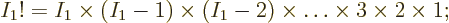there arepos­si­bil­i­ties for the par­ti­cle that comes first in the sorted set, then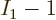pos­si­bil­i­ties left for the par­ti­cle that comes sec­ond, etcetera. Di­vid­ing the ear­lier ex­pres­sion by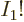, the num­ber of dif­fer­ent sets ofpar­ti­cles that can be se­lected for shelf 1 be­comes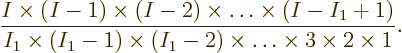But fur­ther vari­a­tions in eigen­func­tions are still pos­si­ble in the way thesepar­ti­cles are dis­trib­uted over the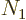sin­gle-par­ti­cle states on shelf 1. There arepos­si­ble sin­gle-par­ti­cle states for the first par­ti­cle of the sorted set, timespos­si­ble sin­gle-par­ti­cle states for the sec­ond par­ti­cle, etcetera, mak­ing a to­tal of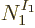vari­a­tions. That num­ber of vari­a­tions ex­ists for each of the in­di­vid­ual sorted sets of par­ti­cles, so the to­tal num­ber of vari­a­tions in eigen­func­tions is the prod­uct: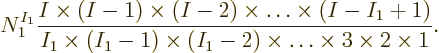This can be writ­ten more con­cisely by not­ing that the bot­tom of the frac­tion is per de­f­i­n­i­tionwhile the top equals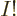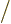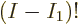: note that the terms miss­ing fromin the top are ex­actly. (In the spe­cial case that, all par­ti­cles on shelf 1, this still works since math­e­mat­ics de­fines 0! = 1.) So, the num­ber of vari­a­tions in eigen­func­tions so far is: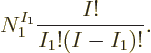The frac­tion is known in math­e­mat­ics as “I choose.

Fur­ther vari­a­tions in eigen­func­tions are pos­si­ble in the way that the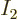par­ti­cles on shelf 2 are cho­sen and dis­trib­uted over the sin­gle-par­ti­cle states on that shelf. The analy­sis is just like the one for shelf 1, ex­cept that shelf 1 has left only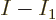par­ti­cles for shelf 2 to chose from. So the num­ber of ad­di­tional vari­a­tions re­lated to shelf 2 be­comes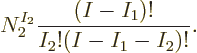The same way the num­ber of eigen­func­tion vari­a­tions for shelves 3, 4, ... can be found, and the grand to­tal of dif­fer­ent eigen­func­tions is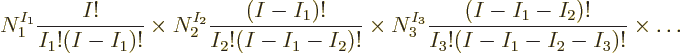This ter­mi­nates at the shelf num­ber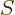be­yond which there are no more par­ti­cles left, when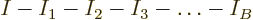0. All fur­ther shelves will be empty. Empty shelves might just as well not ex­ist, they do not change the eigen­func­tion count. For­tu­nately, there is no need to ex­clude empty shelves from the math­e­mat­i­cal ex­pres­sion above, it can be used ei­ther way. For ex­am­ple, if shelf 2 would be empty, e.g.0, then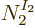1 and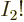1, and the fac­torsand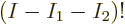can­cel each other. So the fac­tor due to empty shelf 2 be­comes mul­ti­ply­ing by one, it does not change the eigen­func­tion count.

Note that var­i­ous fac­tors can­cel in the eigen­func­tion count above, it sim­pli­fies to the fi­nal ex­pres­sion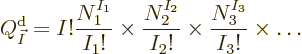Math­e­mati­cians like to sym­bol­i­cally write a prod­uct of in­dexed fac­tors like this us­ing the prod­uct sym­bol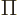: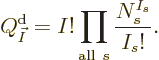It means ex­actly the same as the writ­ten-out prod­uct.

Next the eigen­func­tion count for fermi­ons. Re­fer now to fig­ure 11.3. For any shelf, it is given that there arepar­ti­cles on that shelf, and the only vari­a­tions in eigen­func­tions that can be achieved are in the way that these par­ti­cles are dis­trib­uted over thesin­gle-par­ti­cle eigen­func­tions on that shelf. The fermi­ons are iden­ti­cal, but to sim­plify the rea­son­ing, for now as­sume that you stamp num­bers on them from 1 to. Then fermion 1 can go intosin­gle-par­ti­cle states, leav­ing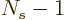states that fermion 2 can go into, then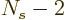states that fermion 3 can go into, etcetera. That pro­duces a to­tal of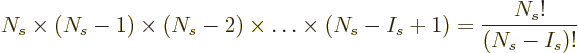vari­a­tions. But most of these dif­fer only in the or­der of the num­bers stamped on the fermi­ons; dif­fer­ences in the num­bers stamped on the elec­trons do not con­sti­tute a dif­fer­ence in eigen­func­tion. The only dif­fer­ence is in whether a state is oc­cu­pied by a fermion or not, not what num­ber is stamped on it. Since, as ex­plained un­der dis­tin­guish­able par­ti­cles, the num­ber of wayspar­ti­cles can be or­dered is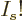, it fol­lows that the for­mula above over-counts the num­ber of vari­a­tions in eigen­func­tions by that fac­tor. To cor­rect, di­vide by, giv­ing the num­ber of vari­a­tions as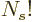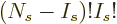, orchoose.” The com­bined num­ber of vari­a­tions in eigen­func­tions for all shelves then be­comes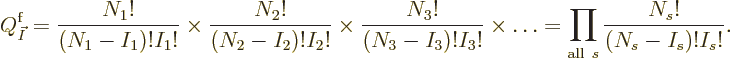If a shelf is empty, it makes again no dif­fer­ence; the cor­re­spond­ing fac­tor is again one. But an­other re­stric­tion ap­plies for fermi­ons: there should not be any eigen­func­tions if any shelf num­beris greater than the num­ber of stateson that shelf. There can be at most one par­ti­cle in each state. For­tu­nately, math­e­mat­ics de­fines fac­to­ri­als of neg­a­tive in­te­ger num­bers to be in­fi­nite, and the in­fi­nite fac­tor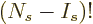in the bot­tom will turn the eigen­func­tion count into zero as it should. The for­mula can be used what­ever the shelf num­bers are.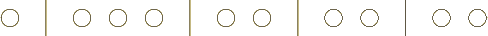Last but not least, the eigen­func­tion count for bosons. Re­fer now to fig­ure 11.2. This one is tricky, but a trick solves it. To il­lus­trate the idea, take shelf 2 in fig­ure 11.2 as an ex­am­ple. It is re­pro­duced in con­densed form in fig­ure D.3. The fig­ure merely shows the par­ti­cles and the lines sep­a­rat­ing the sin­gle-par­ti­cle states. Like for the fermi­ons, the ques­tion is, how many ways can thebosons be arranged in­side thesin­gle-par­ti­cle states? In other words, how many vari­a­tions are there on a schematic like the one shown in fig­ure D.3? To fig­ure it out, stamp iden­ti­fy­ing num­bers on all the el­e­ments, par­ti­cles and sin­gle-state sep­a­rat­ing lines alike, rang­ing from 1 to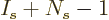. Fol­low­ing the same rea­son­ing as be­fore, there are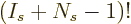dif­fer­ent ways to or­der these num­bered ob­jects. As be­fore, now back off. All the dif­fer­ent or­der­ings of the num­bers stamped on the bosons,of them, pro­duce no dif­fer­ence in eigen­func­tion, so di­vide byto fix it up. Sim­i­larly, all the dif­fer­ent or­der­ings of the sin­gle-par­ti­cle state bound­aries pro­duce no dif­fer­ence in eigen­func­tion, so di­vide by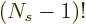. The num­ber of vari­a­tions in eigen­func­tions pos­si­ble by re­ar­rang­ing the par­ti­cles on a sin­gle shelfis then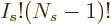. The to­tal for all shelves is